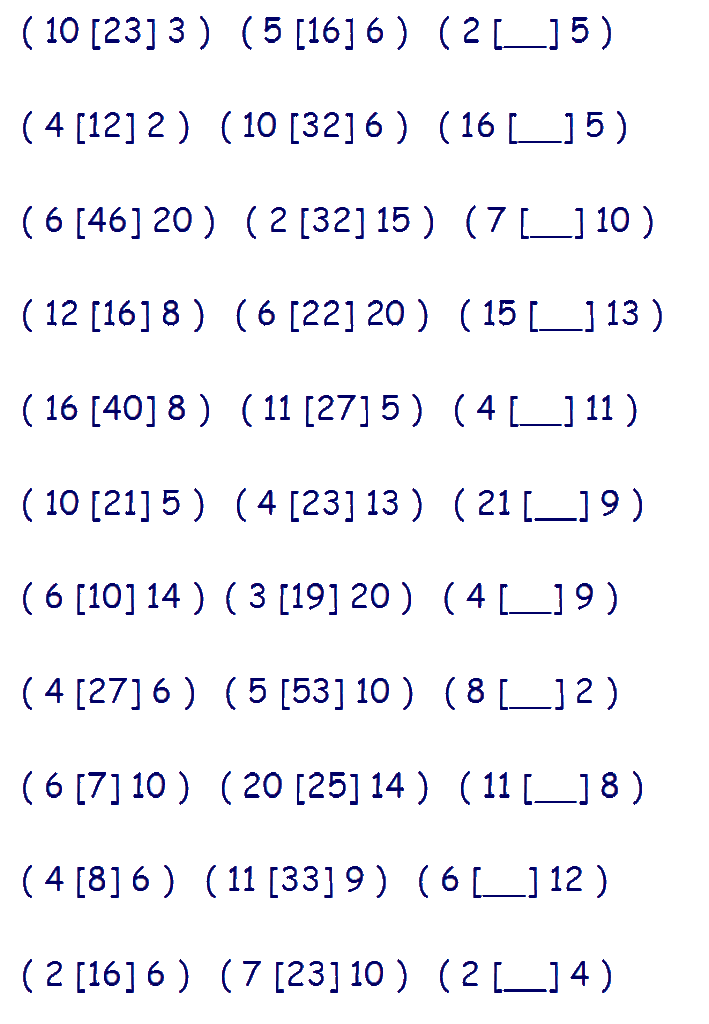# Type K - Number Relationship

With this type of question you are given three groups of three numbers with a number missing from the third group. In each group, the centre number is derived in some way by the outer two numbers. Each group is related in the same way.

You are required to find the missing number from the third group.

Lets look at an example:

## 2  6        5  4        3 [__] 9

Firstly, we need to work out the relationship of the centre number to the outer numbers.

Look at the first group. In this case, what can you do with '2' and '6' to get '12'?

If we multiply 2 by 6, we get 12 - so the rule could be 'multiply the outer numbers'.

Check this with the second group .... 5 x 4 = 20

So now we know the rule, we can use this to discover the missing number from group 3.

3 x 9 = 27 ..... The missing number is 27

## 2  6        5  4        3  9

### Verbal Reasoning Type K - 'Number Relationship' practice